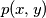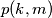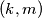### IMO Shortlist 1987 problem 3

Kvaliteta:
Avg: 0,0
Težina:
Avg: 0,0
Does there exist a second-degree polynomial$p(x, y)$ in two variables such that every non-negative integer$n$ equals$p(k,m)$ for one and only one ordered pair$(k,m)$ of non-negative integers?

Proposed by Finland.
Izvor: Međunarodna matematička olimpijada, shortlist 1987